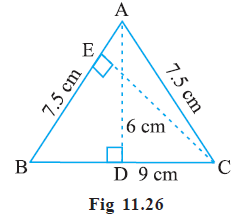# Ex.11.2 Q8 Perimeter and Area - NCERT Maths Class 7

Go back to  'Ex.11.2'

## Question

$$\triangle ABC$$ is isosceles with $$AB = AC = 7.5 \,\rm cm$$ and $$BC = 9 \,\rm cm$$ (Fig 11.26). The height $$AD$$ from $$A$$ to $$BC$$, is $$6 \,\rm cm$$. Find the area of $$\triangle ABC$$. What will be the height from $$C$$ to $$AB$$ i.e., $$CE\,?$$Video Solution
Perimeter And Area
Ex 11.2 | Question 8

## Text Solution

What is known?

$$\triangle ABC$$ is isosceles with $$AB = AC = 7.5 \,\rm cm$$ and $$BC = 9 \,\rm cm$$. The height $$AD$$ from $$A$$ to $$BC,$$ is $$6 \,\rm cm.$$

What is unknown?

The area of $$\triangle ABC$$ and the height from $$C$$ to $$AB$$ i.e., $$CE.$$

Reasoning:

First, find the area of the given triangle using base as $$9 \,\rm cm$$ and height as $$6\,\rm cm.$$ Now, the area of triangle is known, take $$AB = 7.5 \,\rm cm$$ as the base and find height $$CE$$ using the formula of area of triangle.

Steps:

In $$\triangle ABC$$, $$AD = 6 \,\rm cm$$ and $$BC = 9\,\rm cm$$

Area of triangle $$ABC$$  $$=$$ $$\frac{1}{2}$$ Base  $$\times$$ Height

\begin{align}& = \frac{1}{2} \times {\rm{BC}} \times {\rm{AD}}\\&= \frac{1}{2} \times 9 \times 6\\&=27\;c{m^2}\end{align}

Now,

Area of triangle $$ABC$$  $$=$$ $$\frac{1}{2}$$ $$AB$$  $$\times$$ $$CE$$

\begin{align}{\rm{27}} &= \frac{1}{2} \times {\rm{7}}{\rm{.5}} \times {\rm{CE}}\\{\rm{CE}} &= \frac{{2 \times {\rm{27}}}}{{{\rm{7}}{\rm{.5}}}}\\{\rm{CE}} &= \frac{{54}}{{{\rm{7}}{\rm{.5}}}}\\{\rm{CE}} &= 7.2\;\rm{cm}\end{align}

Learn from the best math teachers and top your exams

• Live one on one classroom and doubt clearing
• Practice worksheets in and after class for conceptual clarity
• Personalized curriculum to keep up with school